## Blog

### How to estimate mesh convergence just by looking at stress contour plot

A mesh convergence study is performed to make sure that element sizes are sufficiently small such that the solution obtained using finite element analysis is accurate to a desired level. There are different strategies used to evaluate mesh convergence as discussed in the previous blogs. In this blog I am going to describe how to assess mesh convergence just by looking at unaveraged stress contour plot.

By default, commercial FEA software show the smoothed/averaged stress value at the nodes shared by multiple elements. Such a plot usually appears smooth but it does not present a true picture. In contrast unaveraged stress contour plots might not appear smooth but give more information than just showing the calculated stresses. These plots are a helpful tool to evaluate if the mesh density is sufficient for the given problem. In other words, mesh convergence can be judged by looking at unaveraged stress contour plot. If the unaveraged stresses are not continuous at element boundaries, it can be assumed that the mesh is coarse. If you are not familiar with the ‘averaged’ and ‘unaveraged’ stress terms, you do not need to worry. These terms will be explained soon with the help of illustrations. First we will consider an example to see how to evaluate mesh convergence by looking at stress contour plot.

Now consider a thick cylinder shown in the Figure 1. By taking advantage of the symmetry, only 45-degree sector of the cylinder will be analyzed. Symmetry boundary conditions will be applied on the symmetric edges of the sector. The cylinder is subjected to an internal pressure.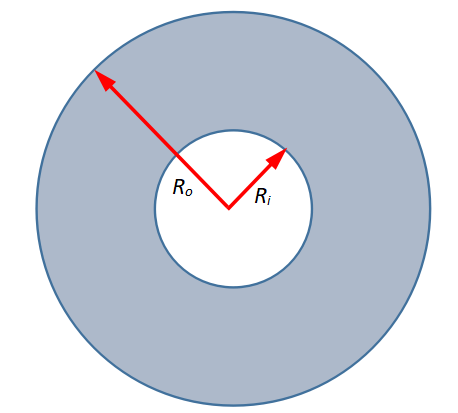Figure 1: Schematic illustration of thick cylinder

The contour plot of unaveraged von Mises stresses is shown in the Figure 2 when an analysis is performed using the coarse mesh. It can be seen that stresses are discontinuous at element boundaries along the radial direction.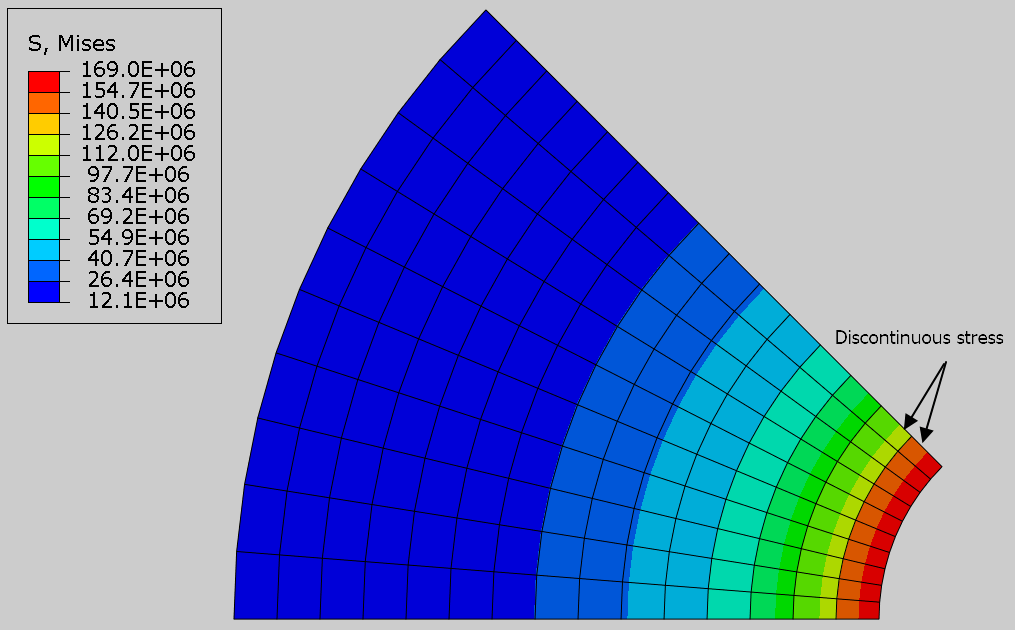Figure 2: Contour plot of von Mises stress for coarse mesh without averaging at nodes

The contour plot of von Mises stresses for this analysis is shown in the Figure 3 when values are averaged at nodes. It can be seen that stresses appear continuous at element boundaries along the radial direction.Figure 3: Contour plot of von Mises stress for coarse mesh with averaging at nodes

The contour plot of unaveraged von Mises stresses is shown in the Figure 4 when an analysis is performed using the refined mesh. It can be seen that stresses are continuous at element boundaries along the radial direction.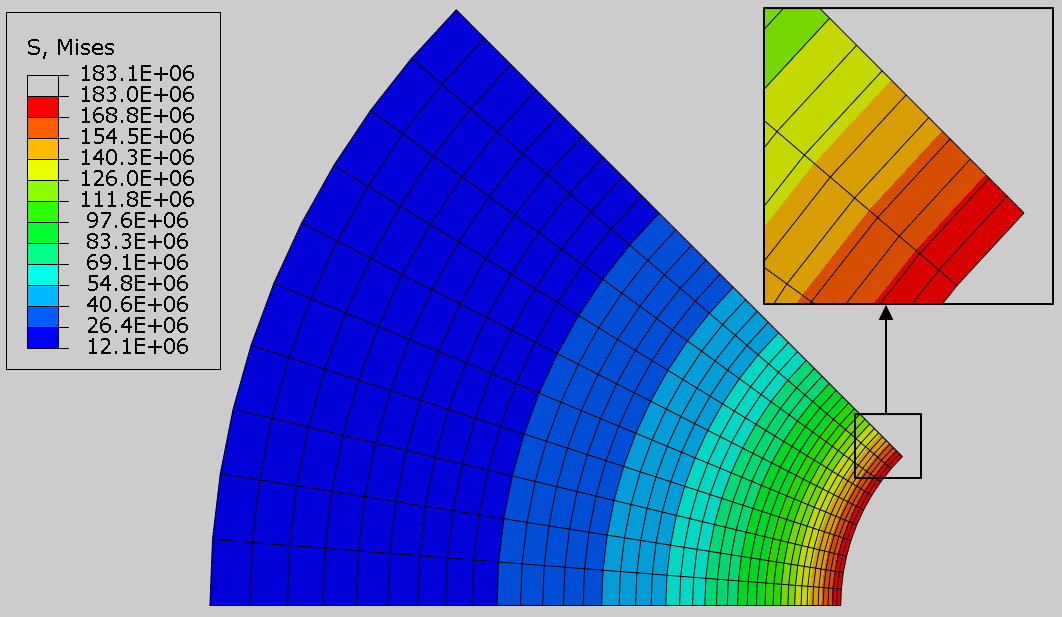Figure 4: Contour plot of unaveraged von Mises stresses for refined mesh

So for a given case of stress distribution, unaveraged contour plot of stress is a valuable tool to evaluate convergence. If the unaveraged stresses are not continuous at element boundaries, it can be assumed that the mesh is coarse and needs to be refined for accurate results. In the Figure 5, contour plot of von Mises stress for a general case is shown with and without averaging of stresses. The stresses appear discontinuous at element boundaries when they are not averaged. The contour plot appears smoother when the stress values are averaged at nodes. By looking at the contour plot for unaveraged stresses, it can be concluded that mesh needs to be refined in the region where plot is discontinuous.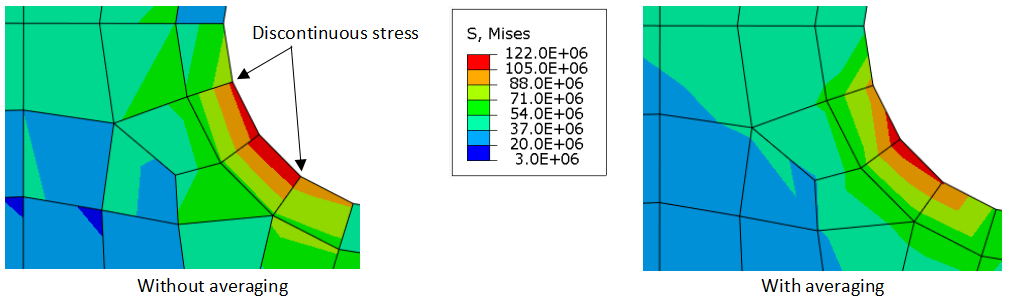Figure 5: Contour plot of von Mises stress with and without averaging for a general case

### Averaged vs unaveraged stresses

A finite element analysis computes the stresses at integration points in each element. Hence the stress values are most accurate at the integration points. These stress values are then extrapolated to find the values at element nodes. For a node shared by multiple elements, there are more than one values which could be associated with that particular node. To associate a single value to the shared node, a smoothed value is calculated by taking an average of extrapolated values of each element. Figure 6 shows a square plate meshed with four elements with a node in the center which belongs to all elements. Each element has four integration points where stress values are computed by FE analysis. The extrapolated values of stress from each element could be significantly different from each other at the center node. The question arises which value should be associated to the center node? To get a single value which could be associated to the center node, an average of stress values is computed. For a sufficiently refined mesh the extrapolated values should be equal or very close to each other. In other words, the solution should be continuous over the elements for a refined mesh. The jump/discontinuity in the stresses are an indication of coarse mesh. This averaged stress value is what makes the contour plot smoother when averaged stress option is used during postprocessing.Figure 6: Schematic illustration of stress extrapolation from integration points to the center node

To elaborate further consider a beam as shown in Figure 7. The beam is fixed on the left side and a load is applied to the right edges as shown in the figure.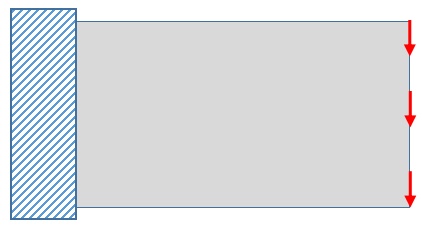Figure 7: Beam geometry with boundary conditions

The Figure 8 shows the mesh generation for the beam. We will focus on first four elements and see how the averaged stress is computed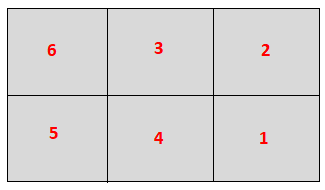Figure 8: Mesh with element numbers for beam

First the FE solver computes the stresses at integration points which are shown in the Figure 9a. To plot the stress contours, these values are then extrapolated to find the stress values at element nodes. The extrapolated values are shown in the Figure 9b. At the center node which is shared by four surrounding elements, there are four different values which could be associated with it. It can be seen that these values differ from each other considerably. To resolve the conflict, an average of extrapolated stress values is computed [ (6.95+6.95+22.68+22.68)/4=14.815] which is associated to this node. In the same way an average stress is calculated at nodes shared by two or more element.Figure 9: Computed stress values at integration points and extrapolated stress values at element nodes

In the Figure 10 contour plot of stress for these four elements is shown with and without averaging of stresses at shared nodes. It can be seen that stress contour appears very smooth when the averaged stress values are considered. This shows that averaging of stresses gives a nice plot but a true picture is obtained when stress plot is seen without averaging of stress values.Figure 10: Contour plot of von Mises stress with and without averaging

### Conclusion

Mesh density affects the accuracy of predictions made by a finite element analysis significantly. Ignoring the mesh convergence´can lead the design process in wrong direction. Although error indicators can be requested to evaluate the mesh convergence, the unaveraged stress contour plot could be used as an alternative in cases where error indicator is not available due to software capability or simply because an analyst forgets to request error output variable.

If you want to learn adaptive meshing in depth, you could have a look at the online course “Solving Non-linear Problems with Abaqus